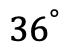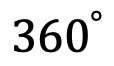# Area of a Sector: Geometry

Hi math friends, has anyone been cooking more during quarantine?  We all know there is more time for cookin’ and eatin’ cakes but have you ever been curious about the exact amount of cake you are actually eating?! Well, you’re in luck because today we are going to go over how to find the area of a piece of cake, otherwise known as the Area of a Sector!

Now, we’ll all be able to calculate just how much we are overdoing it on that pie! Hopefully, everyone is eating better than I am (I should really calm down on the cupcakes).  Ok, now to our question:

*Also, If you haven’t done so, check out the video that goes over this exact problem, and don’t forget to subscribe!

## Explanation:

How do I answer this question?

We must apply/adjust the formula for the area of a circle to find the area of the blue shaded region otherwise known as the sector of this circle.

How do we do this?

Before we begin let’s review the formula for the area of a circle. Just a quick reminder of what each piece of the formula represents:

Step 1: Now let’s fill in our formula, we know the radius is 5, so let’s fill that in below:

Step 2: Ok, great! But wait, this is for a sector; We need only a piece of the circle, not the whole thing.  In other words, we need a fraction of the circle. How can we represent the area of the shaded region as a fraction?

Well, we can use the given central angle value,, and place it over the whole value of the circle,. Then multiply that by the area of the entire circle. This will give us the value we are looking for!

Step 3: Multiply and solve!

Ready for more? Try solving these next few examples on your own to truly master area of a sector!

## Practice Questions:

Find the area of each shaded region given the central angle and radius for each circle:

## Solutions:

What do you think of finding the area of sector? Are you going to measure the area of your next slice of pizza?  Do you have any recipes to recommend?  Let me know in the comments and happy calculating! 🙂

Looking for more about circles? Check out this post on the circle formula here!

## Circle Theorems and Formulas:

Central Angle Theorem

Intersecting Secants Theorem

Inscribed Angles and Intercepted Arc

Circle Theorems